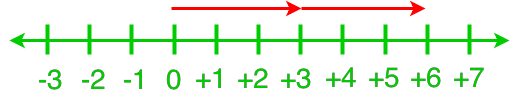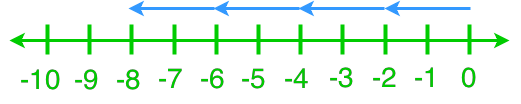# Multiplying integers#### Everything You Need in One Place

Homework problems? Exam preparation? Trying to grasp a concept or just brushing up the basics? Our extensive help & practice library have got you covered.#### Learn and Practise With Ease

Our proven video lessons ease you through problems quickly, and you get tonnes of friendly practise on questions that trip students up on tests and finals.#### Instant and Unlimited Help

Our personalized learning platform enables you to instantly find the exact walkthrough to your specific type of question. Activate unlimited help now!

0/14
##### Examples
###### Lessons
1. Understanding Multiplication Using a Number Line
Write the multiplication statement for each number line.

1.2.2. Multiplication of 1-digit Integers
Use a number line to find the product.
1. (+4) × (+4)
2. (+3) × (-8)
3. (-6) × (-2)
3. Multiplication of 2-digit Integers
Estimate. Then calculate.
1. (+18) × (-33)
2. (-21) × (+46)
3. (-37) × (-19)
4. Multiplication of Integers With Different Digits
Solve the following expressions.
1. (+36) × (-5)
2. (-86) × 0
3. (+167) × (+54)
4. (-245) × (-119)
5. Leo has to read 15 books during the school year. If each book has 334 pages, how many pages in total does Leo have to read?
1. Annie had 50 ml of yogurt every morning for the last 7 days. If there is 650 ml of yogurt left in the case, how much yogurt was there 7 days ago?
0%
##### Practice
###### Topic Notes
Continue from the previous section, we will practice more on multiplication of positive and negative integers. We will look at questions involving multiplying integers and number lines. In addition, we will learn how to estimate double digit integer multiplication.
• Any number multiplied by 0 will equal to 0